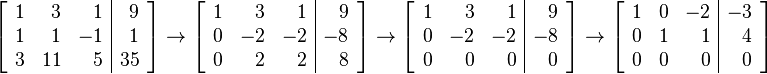## Matrix Gaussian elimination Calculator

 X + Y  +  Z = X + Y  +  Z = X + Y  +  Z =
 Result: X = Y = Z =

In linear algebra, Gaussian elimination is an algorithm for solving systems of linear equations. It is also known as row reduction. It is usually understood as a sequence of operations performed on the associated matrix of coefficients.

For example:Thinkcalculator.com provides you helpful and handy calculator resources.